CBSE Class 12 Sample Paper for 2018 Boards

Class 12
Solutions of Sample Papers and Past Year Papers - for Class 12 Boards

### Find the intervals in which the function f(x) = −3 log (1 + x) + 4 log (2 + x) − 4/(2 + x) is strictly increasing or strictly decreasing.

This is a question of CBSE Sample Paper - Class 12 - 2017/18.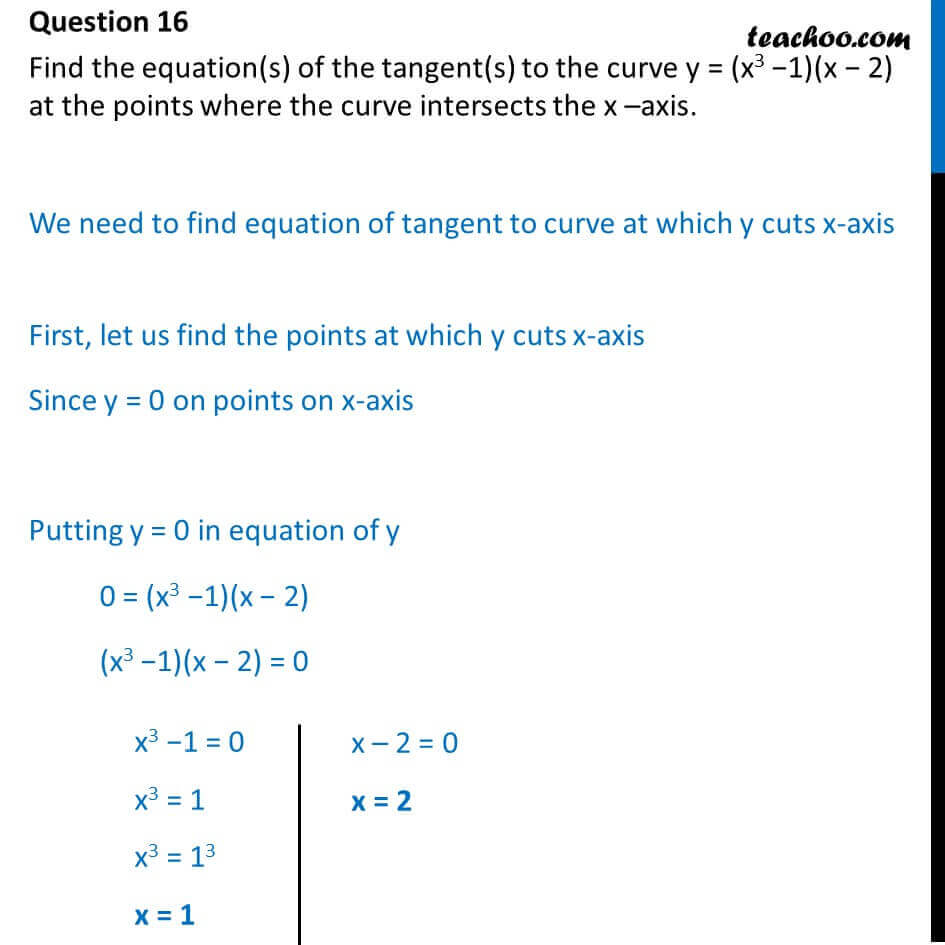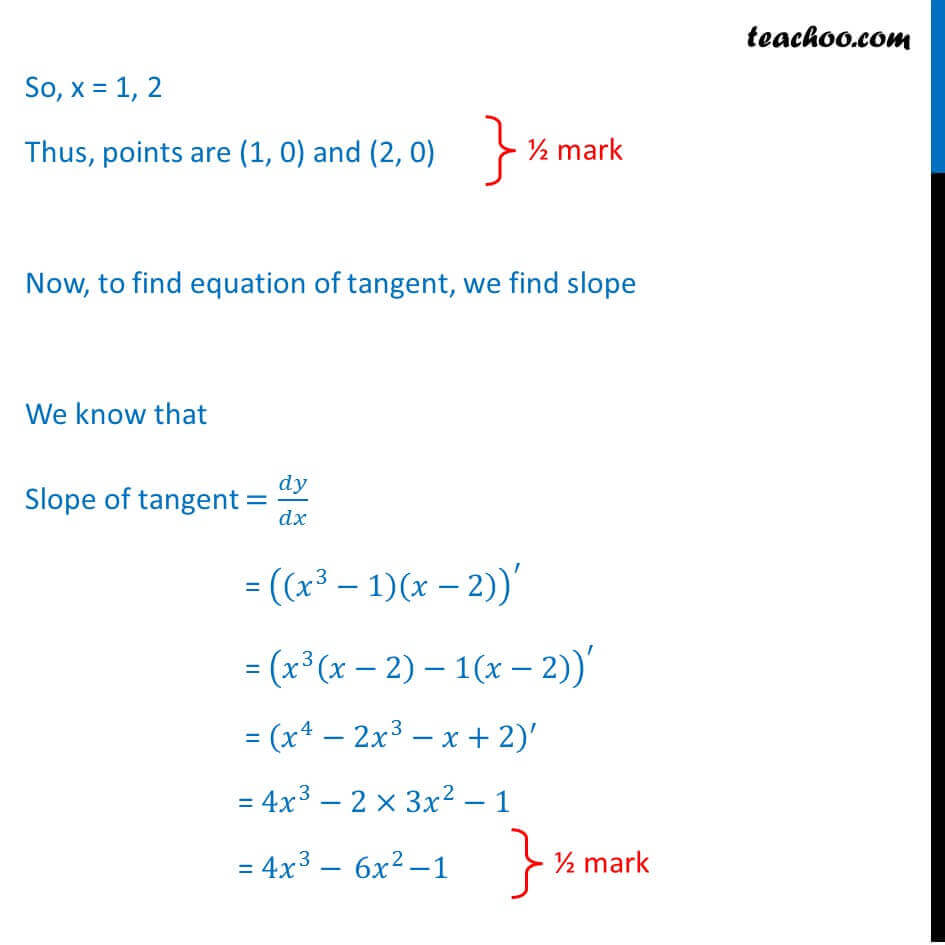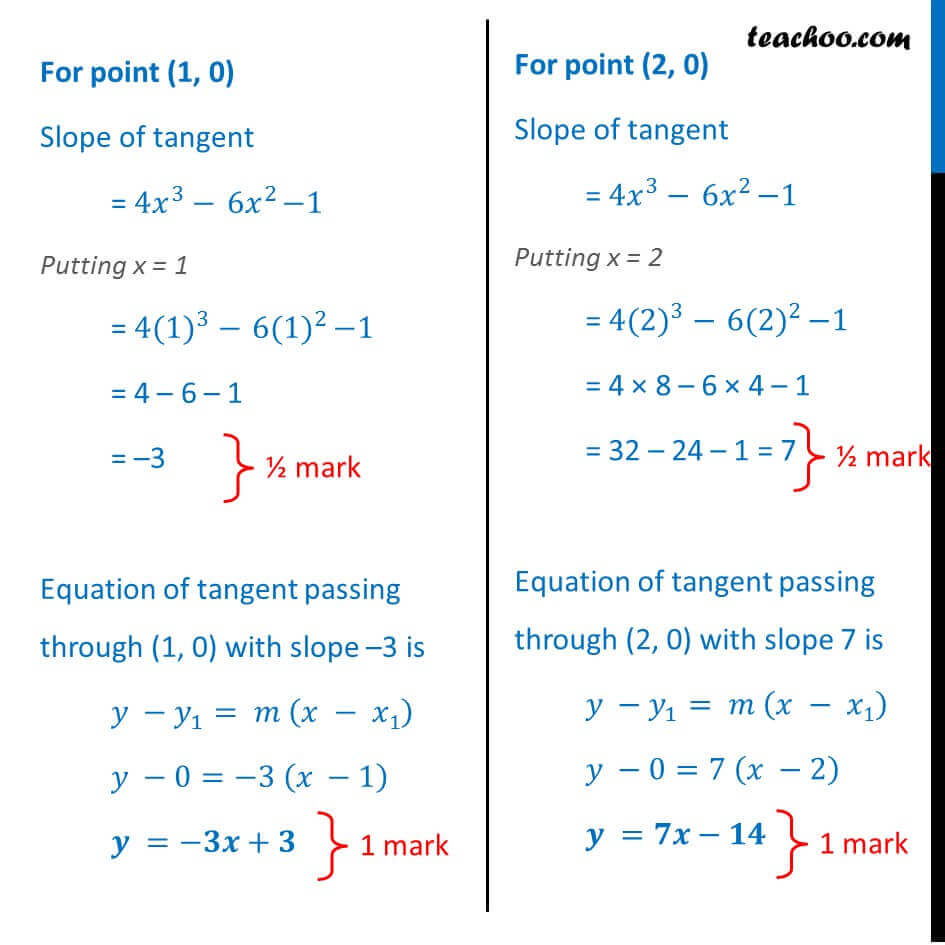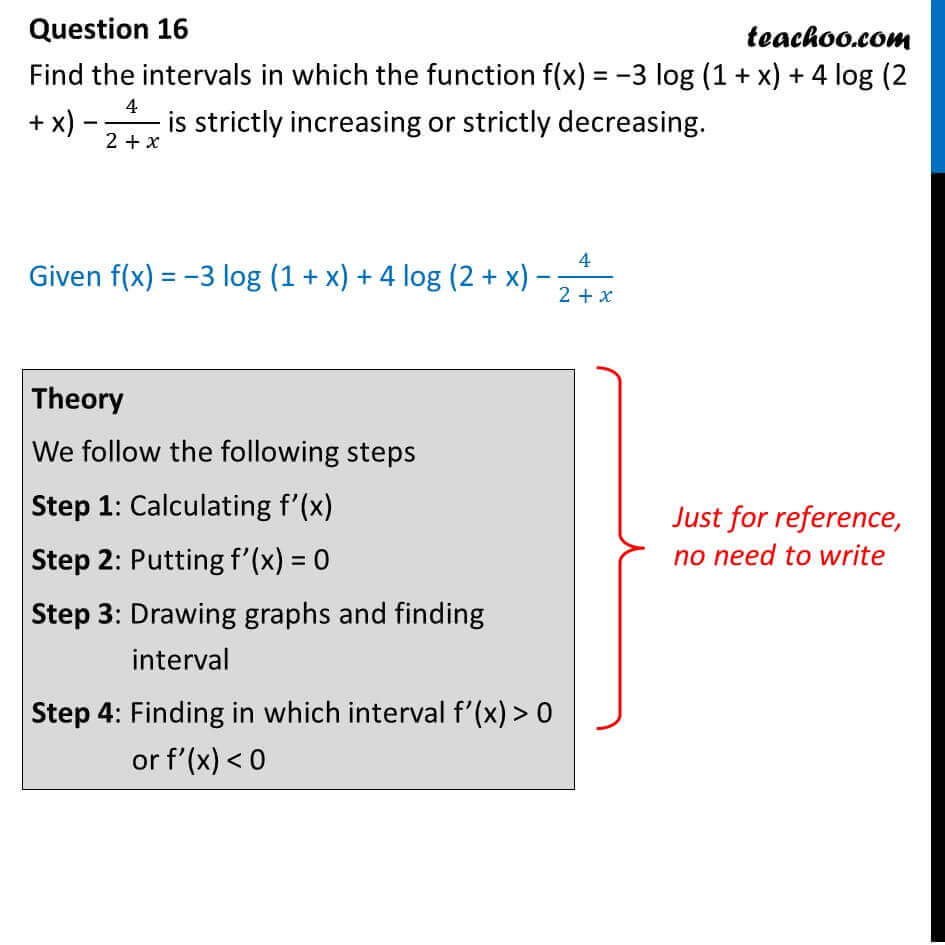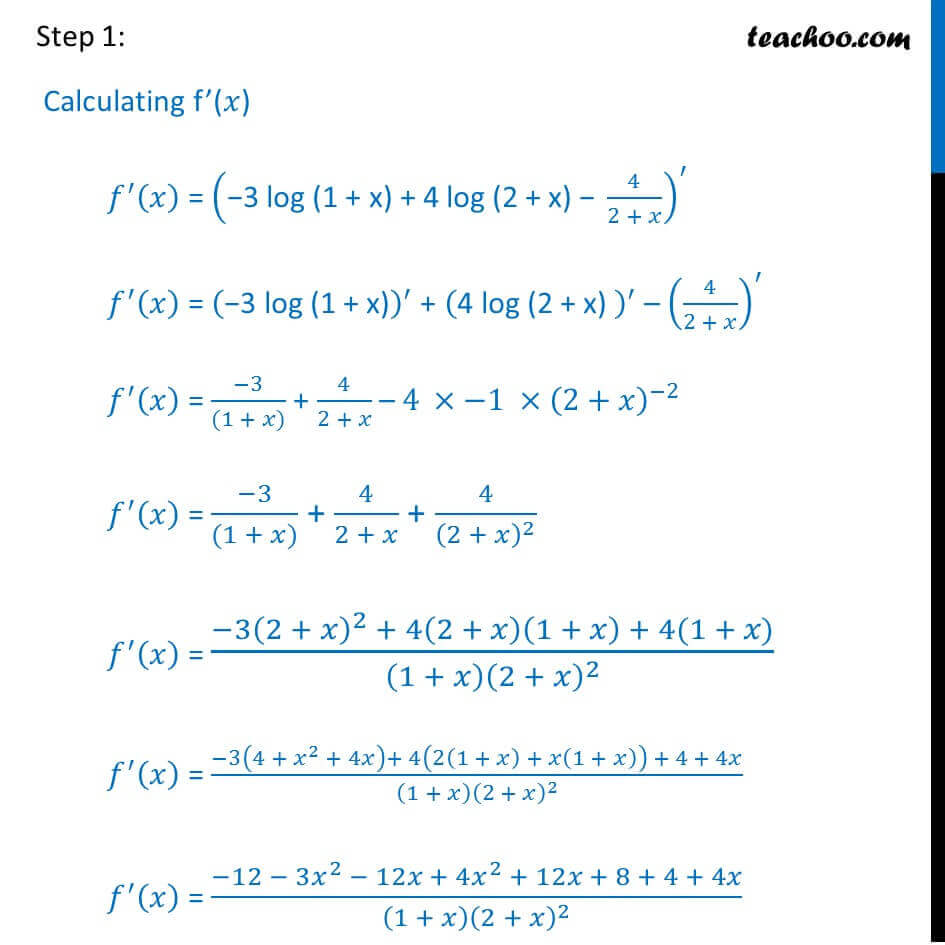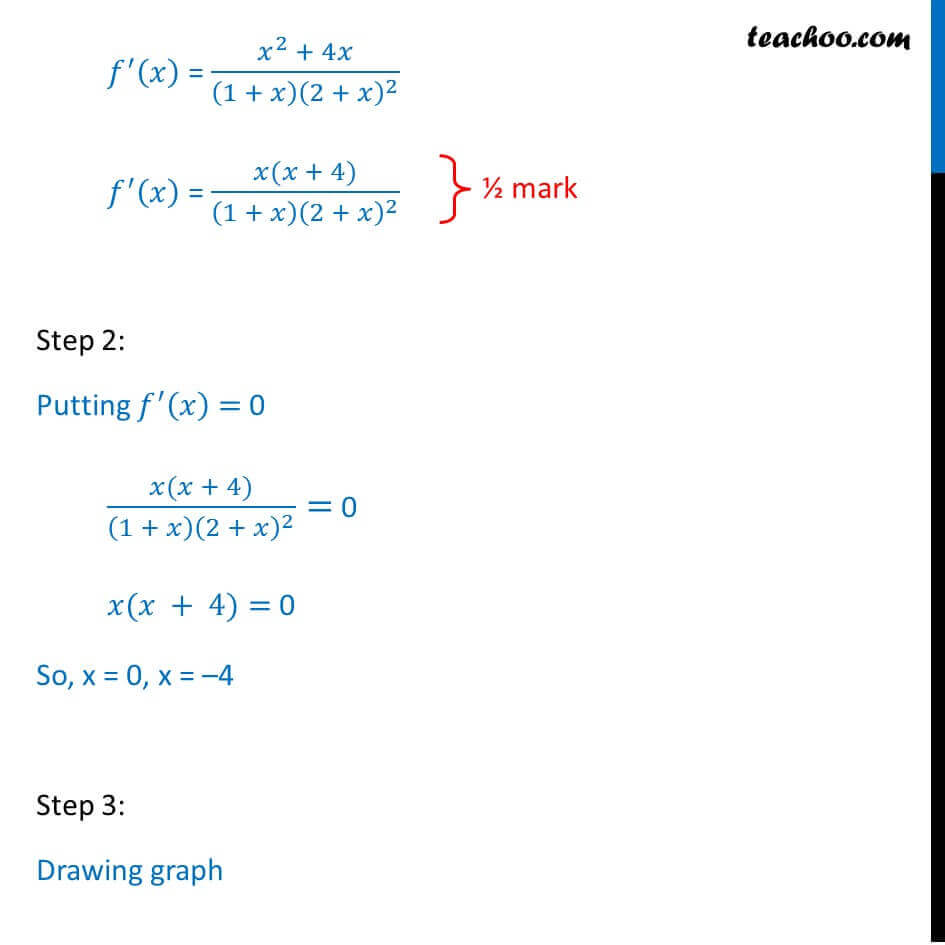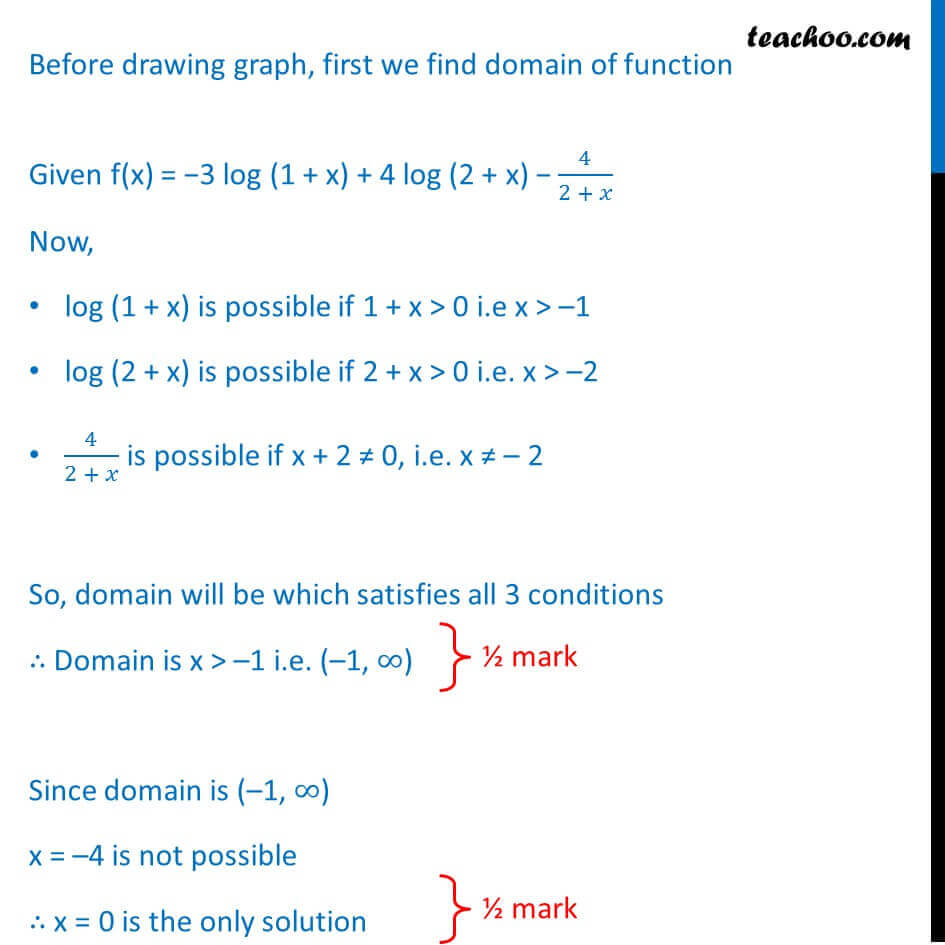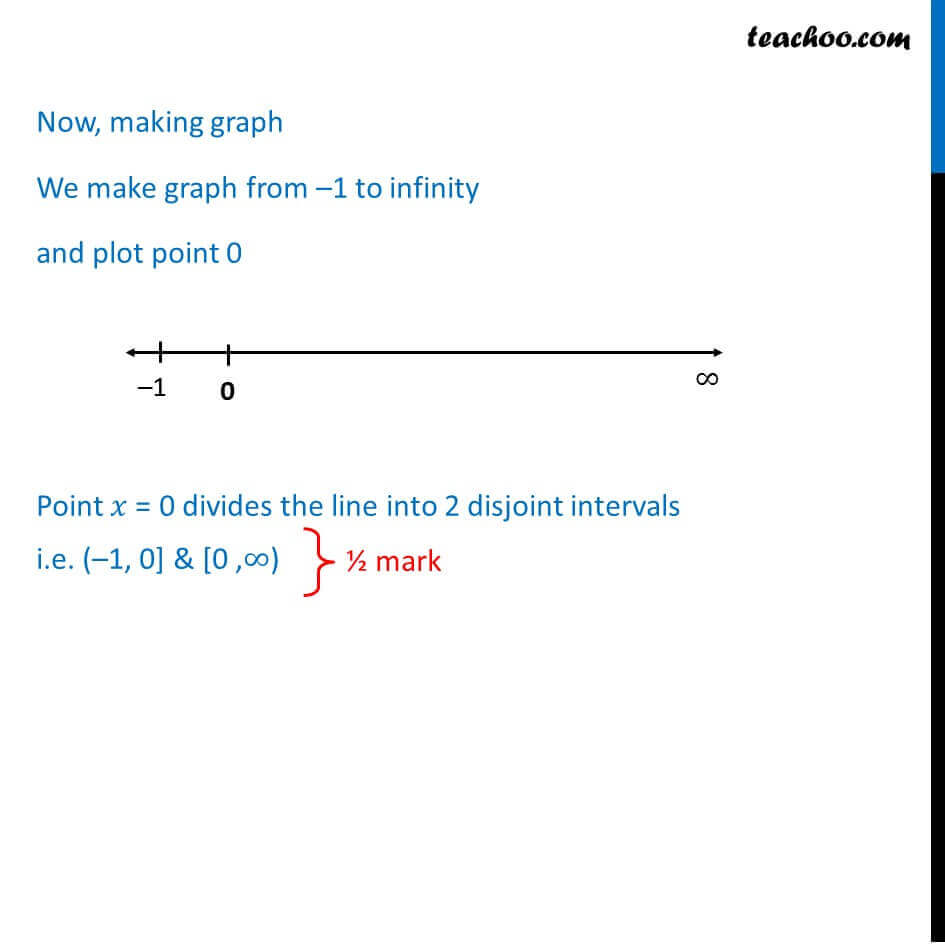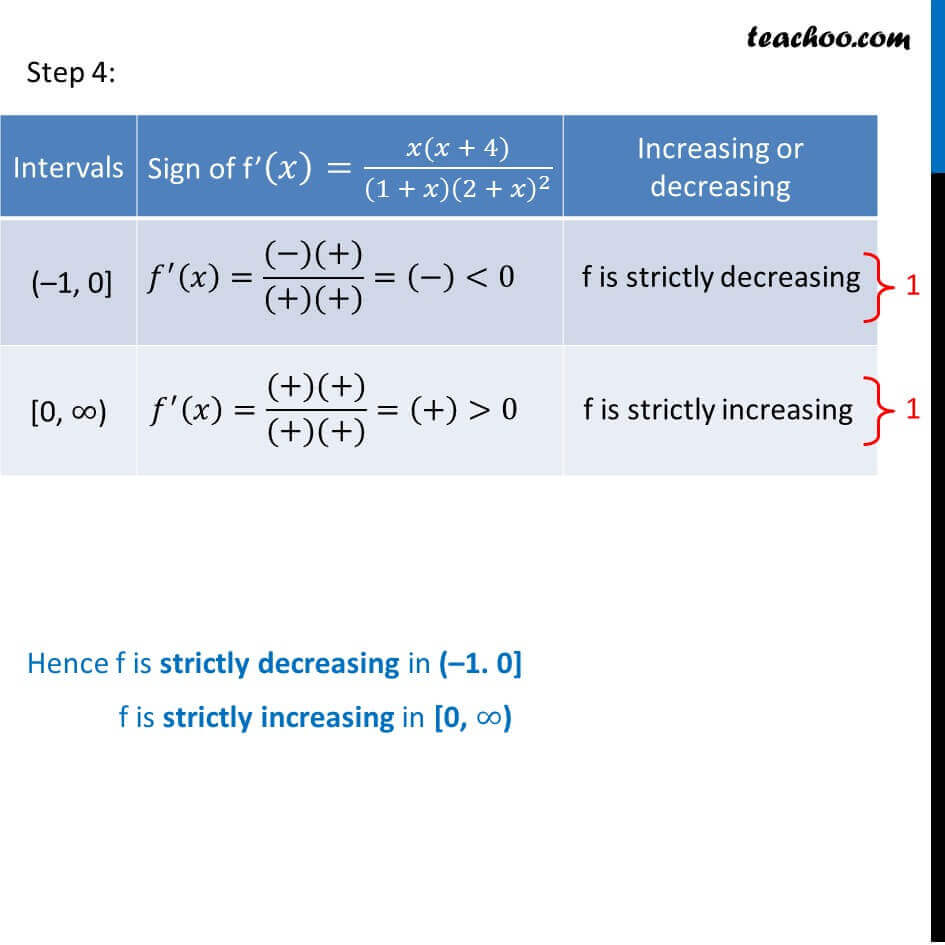Learn in your speed, with individual attention - Teachoo Maths 1-on-1 Class

### Transcript

Question 16 Find the equation(s) of the tangent(s) to the curve y = (x3 1)(x 2) at the points where the curve intersects the x axis. We need to find equation of tangent to curve at which y cuts x-axis First, let us find the points at which y cuts x-axis Since y = 0 on points on x-axis Putting y = 0 in equation of y 0 = (x3 1)(x 2) (x3 1)(x 2) = 0 x3 1 = 0 x3 = 1 x3 = 13 x = 1 x 2 = 0 x = 2 So, x = 1, 2 Thus, points are (1, 0) and (2, 0) Now, to find equation of tangent, we find slope We know that Slope of tangent = / = (( ^3 1)( 2))^ = ( ^3 ( 2) 1( 2))^ = ( ^4 2 ^3 +2)^ = 4 ^3 2 3 ^2 1 = 4 ^3 6 ^2 1 For point (1, 0) Slope of tangent = 4 ^3 6 ^2 1 Putting x = 1 = 4(1) ^3 6(1) ^2 1 = 4 6 1 = 3 Equation of tangent passing through (1, 0) with slope 3 is _1= ( 1) 0= 3 ( 1) = + For point (2, 0) Slope of tangent = 4 ^3 6 ^2 1 Putting x = 2 = 4(2) ^3 6(2) ^2 1 = 4 8 6 4 1 = 32 24 1 = 7 Equation of tangent passing through (2, 0) with slope 7 is _1= ( 1) 0=7 ( 2) = Question 16Theory We follow the following steps Step 1: Calculating f (x) Step 2: Putting f (x) = 0 Step 3: Drawing graphs and finding interval Step 4: Finding in which interval f (x) > 0 or f (x) < 0 Find the intervals in which the function f(x) = 3 log (1 + x) + 4 log (2 + x) 4/(2 + ) is strictly increasing or strictly decreasing. Given f(x) = 3 log (1 + x) + 4 log (2 + x) 4/(2 + ) Step 1: Calculating f ( ) ^ ( ) = (" 3 log (1 + x) + 4 log (2 + x) " 4/(2 + ))^ ^ ( ) = (" 3 log (1 + x)" )^ + ("4 log (2 + x) " )^ (4/(2 + ))^ ^ ( ) = ( 3)/((1 + )) + 4/(2 + ) 4 1 (2+ )^( 2) ^ ( ) = ( 3)/((1 + )) + 4/(2 + ) + 4/(2 + )^2 ^ ( ) = ( 3(2 + )^2 + 4(2 + )(1 + ) + 4(1 + ))/((1 + ) (2 + )^2 ) ^ ( ) = ( 3(4 + ^2 + 4 )+ 4(2(1 + ) + (1 + )) + 4 + 4 )/((1 + ) (2 + )^2 ) ^ ( ) = ( 12 3 ^2 12 + 4 ^2 + 12 + 8 + 4 + 4 )/((1 + ) (2 + )^2 ) Step 2: Putting ^ ( ) = 0 4 3 = 0 4 = 3 = 3/4 ^ ( ) = ( ^2 + 4 )/((1 + ) (2 + )^2 ) ^ ( ) = ( ( + 4))/((1 + ) (2 + )^2 ) Step 2: Putting ^ ( ) = 0 ( ( + 4))/((1 + ) (2 + )^2 )= 0 ( + 4)= 0 So, x = 0, x = 4 Step 3: Drawing graph Before drawing graph, first we find domain of function Given f(x) = 3 log (1 + x) + 4 log (2 + x) 4/(2 + ) Now, log (1 + x) is possible if 1 + x > 0 i.e x > 1 log (2 + x) is possible if 2 + x > 0 i.e. x > 2 4/(2 + ) is possible if x + 2 0, i.e. x 2 So, domain will be which satisfies all 3 conditions Domain is x > 1 i.e. ( 1, ) Since domain is ( 1, ) x = 4 is not possible x = 0 is the only solution Now, making graph We make graph from 1 to infinity and plot point 0 Point = 0 divides the line into 2 disjoint intervals i.e. ( 1, 0] & [0 , )